# SAT Math Multiple Choice Question 994: Answer and Explanation

### Test Information

Question: 994

14.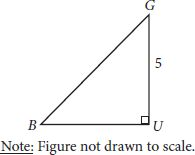Triangle BUG shown is an isosceles right triangle. If the length of side UG is 5 units, what is the sine of ∠G?

• A.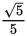• B.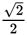• C.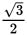• D. 1

Getting to the Answer: An isosceles right triangle is a right triangle that has two legs of equal length, making it a 45-45-90 triangle. This means ∠G is a 45° angle, and the sine of a 45° angle is, which is (B). You should consider memorizing the trig values for common angles (such as 30°, 45°, 60°, 90°, 180°, and 360°) to save yourself some time on Test Day. However, if you didn't recall the value of sin 45°, you could label the triangle using what you know about the side lengths of 45-45-90 triangles (that they are in the ratio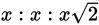or here,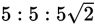), and then use SOH CAH TOA to find that: